# Fractional Divide Frequency Synthesis

## Versal Adaptive SoC Clocking Resources Architecture Manual (AM003)

Document ID
AM003
Release Date
2023-05-16
Revision
1.5 English

The fractional divide in UltraScale and UltraScale+ devices (where the resolution is 0.125) has been replaced in the Versal adaptive SoC by a fractional sigma-delta module (SDM) in the feedback path. The additional 6-bit resolution divider allows generation of finer granularity output clocks than was possible with UltraScale devices. The best jitter performance of an MMCM is obtained when using it in integer mode. When the fractional part of M+F is set to 0, integer mode is automatically chosen. When running the MMCM in fractional mode make sure the VCO runs at the maximum possible frequency for the application. Following examples are provided to highlight the mechanism while the Clock Wizard will automatically deal with this when fractional output frequencies are required. Use attributes for fractional divide:

``CLKFBOUT_FRACT : integer := 0;``
``CLKFBOUT_MULT : integer := 42;``

To put the focus on the fractional divide, the steps to calculate M, D, and other values are omitted from the following examples:

## Example 1

Assume FCLKOUT must be 296.703 MHz for an FCLKIN of 27 MHz.

• D = 10 and M = 109

•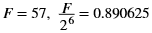•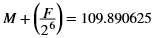• FVCO = 2967.046875 MHz from: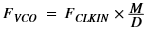• FCLKOUT is then 296.7046875 MHz (rounded to 296.705 MHz)

The previous calculation does not use the highest possible VCO frequency to generate the output clock frequency of 296.703 MHz. Recalculate for the highest possible VCO frequency.

• D = 14 and M = 153

•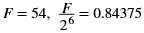•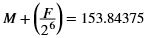• FVCO= 4153.78125 MHz
• FCLKOUT is then 296.69866 MHz (rounded to 296.699)

The first calculation can achieve 296.7046875 MHz and the second can achieve 296.69866 MHz. Both settings are slightly off from the target frequency of 296.703 MHz, which is due to the resolution of the fractional mode. To improve the frequency error, higher reference frequencies must be used.

## Example 2

Assume FCLKOUT must be 335 MHz for an FCLKIN of 30 MHz
• D = 1, M = 100, and O = 9

•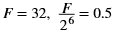•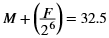• FVCO= 3015 MHz from: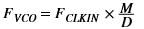FCLKOUT is then 335 MHz

## Example 3

•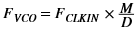•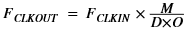• Desired FCLKOUT is 212.3457 MHz for an FCLKIN of 50 MHz
• FVCOmax = 4320 MHz
• The highest possible VCO frequency is obtained for a O value of: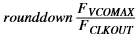• rounddown(3420/212.3457) = 20

•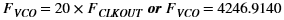•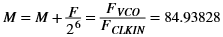• Assume D = 1

•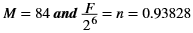•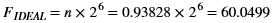• F must be an integer value, therefore take the rounddownFIDEAL or F = 60

Redo the calculations to get the real FCLKOUT: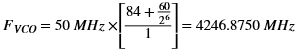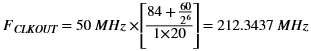212.3457 MHz was required.